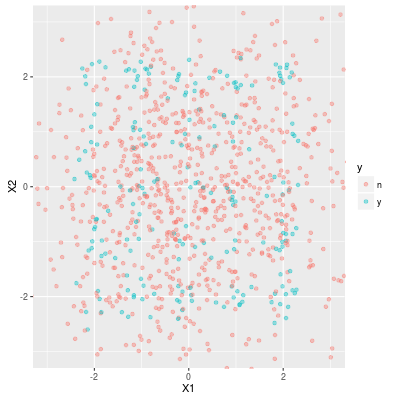Data Experiment #07-1 Imbalanced classification problems

## Imbalanced classification problems

A skewed class is a binary variable which takes hardly one of the values. For example 99% of the values are 0 and the rest is 1. When we train a statistical model for such a skewed class, the following problems always arise:

• The model "the target value is always 0" achieves a very high accuracy, even though the model is absolutely useless.
• Any (simple) statistical models do not defeat the useless model or are a very similar model to it.

When we try to construct a statistical model for a skewed class, the accuracy of the model is hardly most important. For example we want to find (relatively small number of) samples which are likely 1. Then we may allow that there are samples with 0 in the picked samples.

Even if the goal of a statistical model which we create is not the accuracy, a simple statistical model does not help. A sampling method is one of the methods for such a situation. The aim of this and the following entries is to examine several sampling methods and to observe what happens when applying a sampling method.

NB: A cost-sensitive learning is also known as a method for an imbalanced classification. But we do not try it this time. For a comparison between a cost-sensitive learning and a sampling method you should consult Weiss–McCarthy–Zabar.

## An example of an imbalanced classification problem

Our data look like the followingAround 20% of the data are positive (y). By the definition the positive samples lie around lattice points. You may easily find the pattern. More importantly, the target variable does not seem so badly skewed.

But simple statistical models do not work well. The following image shows that the scatter plot of our data and the prediction of some models. The blue region are the points which a model predicts as a positive sample.It is obvious that a linear model (elastic net) is not able to pick the positive samples because of the pattern. But SVM also fails to detect the positive samples. That is because that SVM do not fit outliers. While this is an advantage of SVM, the SVM model regards positive samples as outliers in this case.

In comparison with SVM, the ensemble tree models (i.e. random forest and XGBoost) seems to pick successfully positive samples partly. But the performance of the models is not so good.

     method accuracy y.rate    recall precision  f1.score
1     all.n    0.783  0.000 0.0000000 0.0000000 0.0000000
2    glmnet    0.783  0.000 0.0000000       NaN       NaN
3 svmRadial    0.783  0.000 0.0000000       NaN       NaN
4        rf    0.763  0.150 0.2995392 0.4333333 0.3542234
5   xgbTree    0.800  0.109 0.2903226 0.5779817 0.3865031


In the above table all.n is the "useless" model, that is it predicts that all samples are negative. Even though the XGBoost model achieves a higher accuracy than the useless model, it can pick only 30% of positive samples.

## Next Entry

In the next entry we apply several sampling methods to a famous data set (rather than an artificial data set).

### Note

In this series of entries, we do not tune meta parameters explicitly. Namely we let the caret package tune the parameters. We should always tune carefully the parameter so that the performance on a validation set is maximum. But the aim of this series is for understanding sampling methods and behavior of several statistical learning after the sampling. Therefore we concentrate on sampling methods and we rely on caret for turning parameters.

Categories: #data-mining